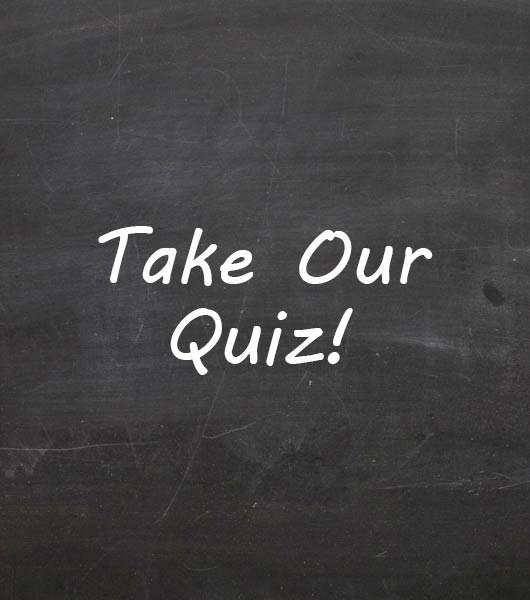10 Questions | Total Attempts: 64Settings• 1.
2.236067… is a rational number
• A.

True

• B.

False

• 2.
-|-5|=5
• A.

True

• B.

False

• 3.
3/4 < 1/4
• A.

True

• B.

False

• 4.
1/3 + 1/5 =
• A.

11/15

• B.

8/15

• C.

2/15

• D.

2/8

• 5.
According to pythagorean theorem a=3, b=4, c=?
• A.

5

• B.

7

• C.

1

• D.

25

• 6.
15/3 is a irrational number
• A.

True

• B.

False

• 7.
A natural number is also a whole number
• A.

True

• B.

FalseBack to top